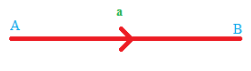# Vector And Scalar Quantities

In mathematics and physics, we have physical quantities which can be categorized in two ways, namely

• Scalar Quantity
• Vector Quantity

In this article, let us discuss what are vector and scalar quantities with examples.

## Scalar Quantity Definition

The physical quantities which have only magnitude are known as scalar quantities. It is fully described by a magnitude or a numerical value. Scalar quantity does not have directions. In other terms, a scalar is a measure of quantity. For example, if I say that the height of a tower is 15 meters, then the height of the tower is a scalar quantity as it needs only the magnitude of height to define itself. Let’s take another example, suppose the time taken to complete a piece of work is 3 hours, then in this case also to describe time just need the magnitude i.e. 3 hours.

### Scalar Quantity Examples

Other examples of scalar quantities are mass, speed, distance, time, energy, density, volume, temperature, distance, work and so on.

## Vector Quantity Definition

The physical quantities for which both magnitude and direction are defined distinctly are known as vector quantities. For example, a boy is riding a bike with a velocity of 30 km/hr in a north-east direction. Then, as we see for defining the velocity, we need two things, i.e. the magnitude of the velocity and its direction. Therefore, it represents a vector quantity.

### Vector Quantity Examples

Other examples of vector quantities are displacement, acceleration, force, momentum, weight, the velocity of light, a gravitational field, current, and so on.

## Difference Between Scalar and Vector Quantity

Let us discuss some difference here:

 Difference Between Scalar and Vector Quantity Scalar Quantity Vector Quantity It has magnitude only It has both magnitude and the direction It does not have direction It has direction It is specified by a number and a unit It is also specified by a number along with the direction and unit It is represented by quantity symbol It is represented by quantity symbol in bold or with the arrow sign above Example: Temperature, speed, etc Example: Acceleration, velocity, etc

## Vector Representation

Let us have a look at the line segment drawn below. A vector quantity always has a starting point and an endpoint. The two endpoints of the given line segment are distinguishable as and. It represents a directed line segmentThe directed line segment with an initial point  A and terminal point B  is symbolically denoted as  AB  in bold or as

$$\begin{array}{l}\overrightarrow{AB}\end{array}$$
and the length a of the vector represents its magnitude which is denoted by |AB|. Instead of using double letter notation we can use a single letter notation to represent a vector as a, b, c and it denotes their magnitudes. As it is difficult to write letters in bold we use a bar above the letters to represent vectors as
$$\begin{array}{l}\overline{a}\end{array}$$
.

Therefore, if

$$\begin{array}{l} \overrightarrow{AB} = a \end{array}$$
then
$$\begin{array}{l} |\overrightarrow{AB}| = a \end{array}$$
where
$$\begin{array}{l}|\overrightarrow{AB}|\end{array}$$
indicates the magnitude of the vector. Also, the magnitude is called the modulus.

### Characteristics of Vectors

The characteristics of the vectors are as follows:

• Vectors possess magnitude as well as the direction
• It does not obey the ordinary law of algebra
• Either the magnitude or direction change or both change

### Scalar and Vector Quantity Example

Question:

Find out the scalar and vector quantity from the given list.

Force, Speed,  Electric field, Angular Momentum, Magnetic Moment, Temperature, Linear Momentum, Average Velocity.

Solution:

From the given list,

• Scalar Quantities – Speed, Temperature.
• Vector Quantities – Force, Electric field, Angular Momentum, Magnetic Moment, Linear Momentum, Average Velocity.

Now we are familiar with what are vectors and scalars. Now if somebody asks if acceleration is a vector or a scalar, we can easily tell that it’s a vector because it has direction as well as magnitude. Similarly, when asked if the distance is a vector or scalar, it is quite evident that as distance has only magnitude, it is a scalar quantity.

Keep learning with BYJU’S – The Learning App to learn better.

Test your Knowledge on Vector And Scalar Quantities

#### 1 Comment

1. sambit mishra

The directed line segment with an initial point A and terminal point B is symbolically denoted as AB in bold or as AB−→− and the length a of the vector represents its magnitude which is denoted by |AB|. Instead of using double letter notation we can use a single letter notation to represent a vector as a, b, c and it denotes their magnitudes. As it is difficult to write letters in bold we use a bar above the letters to represent vectors as a¯¯¯.